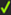﻿ MechDesigner Reference & User Interface > 2: Model-Editor, Mechanism-Editor, Part-Editor > Mechanism-Editor: > Kinematic Function-Blocks > Kinematic FB | Add Point-Data FB

# Kinematic FB | Add Point-Data FB

### Why use a Point-Data Function-Block?

 The Point FB measures motion-values of a Point or Motion-Point with respect to the Mechanism Plane. It has three output-connectors. From top-to-bottom, they are: ➔X-axis motion-values➔Y-axis motion-values➔Magnitude of the motion-values equal to:o√(X2 + Y2)Note: Motion-Values include the Position, Velocity, and Acceleration of the Point or Motion-Point. The Point must be a child to a kinematically-defined Part.

Toolbar :

Kinematics FB toolbarPoint-Data FB

Dialog-Box :

STEP 1: Add the Point-Data Function-Block to the graphic-area

 1.Click Kinematics FB Toolbar > Point-Data FB 2.Click again in the graphic-area

The Point-Data FB is now in the graphic-area.

How to Edit :

STEP 2: Open the Point-Data dialog-box:1.Double-click the Point-Data FB in the graphic-area - or -Point Data dialog
Select a Point:

STEP 3: Select a Point (or Motion-Point)

 When the box is Select a Point: 1.Click a Point in the graphic-area.

The box is now: Selected Point is:

STEP 4: Close the Point-Data FB

 1.Clickin the Point-Data FB

The motion-values for the Point (or Motion-Point) are now at the output-connectors of the Point-Data FB.Point-Data FB
Selected Point is:

Different uses of the Point-Data FB:

You can use the motion-values at the output-connector of a Point-Data FB in three ways:

 •To plot the Position, Velocity and Acceleration of the Point with a Graph FB - see Note 1, below•At the input-connector of a Motion FB. The motion-values from the Point-Data FB become the independent variable, rather than, more typically, the output of a Linear-Motion FB. - see Note 2, below•At the input-connector of a Motion-Dimension FB. The Motion-Part will have the motion as given by output-connector of the Point-Data FB.

Note 1:

To plot the Position, Velocity and Acceleration of the Point in the same graph.

1.Drag a wire from the same output-connector of the Point-Data FB three times to three different input-connectors on a Graph FB,

2.Use the Y–axis display options in the Graph dialog-box to display all motion derivatives.

Note 2:

2 & 3: will give a message in the Feedback-Area: '1 Mechanism dependencies detected'.

You should rebuild the model when the number of dependencies becomes more than 1 if the data is critical, before you use the data for a Cam, for example.